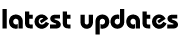Ganit Mantra Series : Circle-1

Ganit Mantra Series : Circles-1 ( An introduction )A  circle is the locus of a point in a plane which moves in such a way that  its distance from a fixed point is always constant. The fixed point is called the centre of the circle while the fixed distance is called the radius of the circle. Let C be  the centre of the circle and the radius by r. For any point P(x, y) lying on the circle, the length PC must be equal to r.

TO KNOW DETAILED CLICK THE ABOVE LINK ….My Great Web page